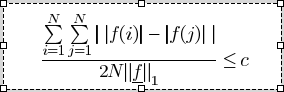# Help: cvx codes about convex

cvx_begin
variable W(1,dimC(3))
minimize(W*normPerSliceDiag*W');
%TotalWNorm = norm(W*normPerSliceDiag,1);
subject to
norm(W,1)<=1;
for RepeatT =1:dimC(3),
for RepeatK =1:dimC(3),
(1)          sum(sum(abs(abs(W(RepeatT)*normPerSlices(RepeatT))-abs(W(RepeatK)*normPerSlices(RepeatK))))) >= 2*GiniMax*dimC(3);
%1+2*sum(abs(W(RepeatT)*normPerSlices(RepeatT))*(dimC(3)-RepeatT+0.5))/(dimC(3)*TotalWNorm)>GiniMax;%%
(2)      % 1 + 2*sum(abs(W(RepeatT)*normPerSlices(RepeatT))*(dimC(3)-RepeatT+0.5)) > 2*GiniMax*(dimC(3)*norm(normPerSliceDiag,1));%%
%(1-2*sum(abs(AB{i})*(dimC(3)-i+0.5)/dimC(3)))>GiniMax;
end
end
cvx_end


the above code says:
(1):Disciplined convex programming error:
Illegal operation: {convex} - {convex}
or
(2):Disciplined convex programming error:
Illegal operation: {convex} - {real constant}

Your problem must be convex. Do you have proof that your model is convex? If not you are likely to use the wrong tool.

This

{convex} - {convex}

most likely means you have something like

f(x)-g(x) <= 0

where both functions are convex, but that does NOT in general specify a convex set. An example is

x^2-y^2 <= 0

As @Erling says
sum(sum(abs(abs(W(RepeatT)*normPerSlices(RepeatT)) - abs(W(RepeatK)*normPerSlices(RepeatK))))) >= 2*GiniMax*dimC(3);
does not follow CVX’s DCP rules, and doesn’t look to me that it can be reformulated as a convex constraint.

Thank you very much! I will check my convex model!

Thank you very much! The model is convex, but I can try it by what you refer to YALMIP

Thank you very much! My model is convex, but it may not satisfy the DCP rulesets!